Next: Scaling Stress Seeds Up: Photon-Baryon Fluid Previous: Entropy and Heat Conduction

## Dissipation

The generation of viscosity and heat conduction in the fluid dissipates fluctuations through the Euler equations with (89) and (94),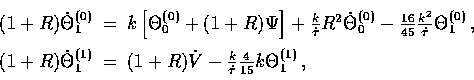where we have dropped the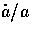factors under the assumption that the expansion can be neglected during the dissipation period. We have also employed Eqn. (85) to eliminate higher order terms in the vector equation. With the continuity equation for the scalars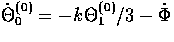(see Eqn. 60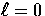, m=0 ), we obtain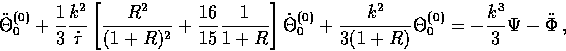(76)
which is a damped forced oscillator equation.

An interesting case to consider is the behavior in the absence of metric fluctuations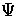,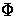, and V. The result, immediately apparent from Eqn. (96) and (97), is that the acoustic amplitude (m=0) and vorticity (m=1) damp as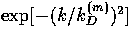where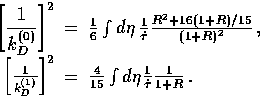Notice that dissipation is less rapid for the vectors compared with the scalars once the fluid becomes baryon dominated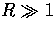because of the absence of heat conduction damping. In principle, this allows vectors to contribute more CMB anisotropies at small scales due to fluid contributions. In practice, the dissipative cut off scales are not very far apart since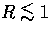at recombination.

Vectors may also dominate if there is a continual metric source. There is a competition between the metric source and dissipational sinks in Eqns. (96) and (97). Scalars retain contributions to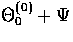of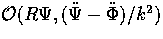(see Eqn. (84) and ). The vector solution becomes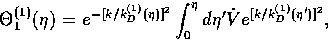(77)
which says that if variations in the metric are rapid compared with the damping then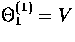and damping does not occur.Next: Scaling Stress Seeds Up: Photon-Baryon Fluid Previous: Entropy and Heat Conduction
Wayne Hu
9/9/1997